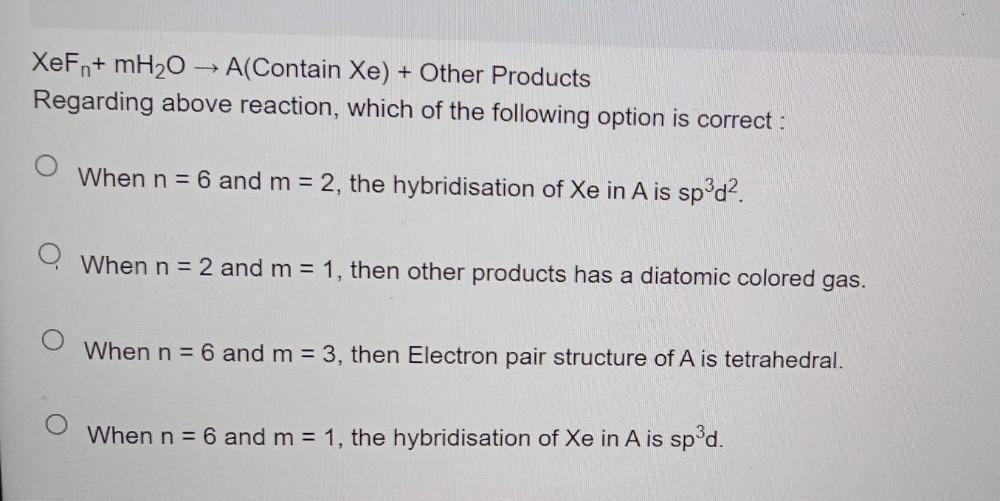Question:

# XeFn+ mH2O → A(Contain Xe) + Other Products Regarding above reaction, which of the following option is correct : O When n = 6 and m = 2, the hybridisation of Xe in A is sp^3d^2. O When n = 2 and m = 1XeFn+ mH2O → A(Contain Xe) + Other Products Regarding above reaction, which of the following option is correct : O When n = 6 and m = 2, the hybridisation of Xe in A is sp^3d^2. O When n = 2 and m = 1, then other products has a diatomic colored gas. O When n = 6 and m = 3, then Electron pair structure of A is tetrahedral. O When n = 6 and m = 1, the hybridisation of Xe in A is sp^3d.